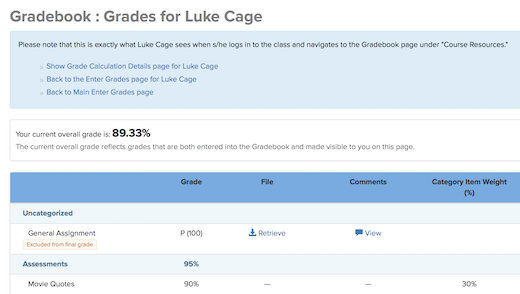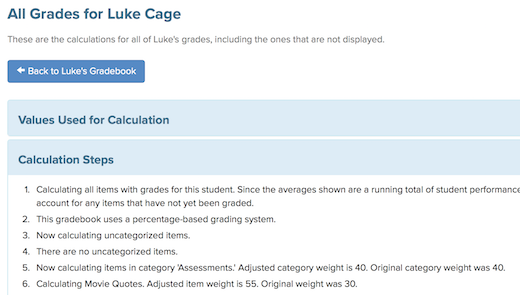# See the Exact Steps Taken in Calculating a Student Grade in the Gradebook

December 13, 2018 | 1 Minute Read

Gradebooks can be complicated things, with many activities grouped into categories and different rules about how individual grades in a category factor into a student's final grade. It can sometimes be difficult to know the exact set of steps taken in calculating a student's grade.

Fortunately, the Gradebook in CoursePlus has a tool for faculty and TAs that shows the exact steps taken in calculating a student's grade in the course Gradebook. This tool can be accessed at any time, even before the final grade in the class is calculated, and the steps shown will update when you add additional scores in to the Gradebook.

To access this tool, go to the student view of any student in the Gradebook. For example, in this example class, we're viewing the Gradebook page for a student named Luke Cage:Click "Show Grade Calculation Details page for [student name]" at the top of the page. This reveals all the steps, in order, that the Gradebook took to calculate the current student grade in the class:Both the actual current grade and the current grade as displayed to the student are shown. Remember that the grade as displayed to the student may be different than the actual, real current grade because sometimes indivdiual graded items aren't displayed to students. For example, you may have graded a midterm, but not displayed grades to students because not all students took the midterm. This is why we show calculations for both the actual current grade, and what is currently shown to students.

We hope that the information shown in this tool will help you better understand the exact steps taken to calculate a student grade in a course. You can even share this information with students who may have concerns about how their grade is calculated.